Function Repository Resource:

# GraphCoordinationSequence

Find the number of vertices at each distance in a symmetric graph

Contributed by: Ed Pegg Jr
 ResourceFunction["GraphCoordinationSequence"][g] finds the number of vertices at distance k for each vertex in the graph g and returns the distinct behaviors.

## Details

In a square lattice, the coordination sequence starts 1, 4, 8, 12, 16, 20, 24, ..., with multiples of 4 following. By the taxicab metric, there is one lattice point at distance 0, 4 at distance 1, and so on.
Usually, the coordination sequence is only of interest when it is the same for all vertices.
For a disconnected graph, the number of unreachable points is placed after ∞.
Finding the coordination sequence for a specific graph is compuationally easy. Finding the graph for a specific coordination sequence is extremely difficult. Whether a graph with coordination sequence {1, 57, 3192} exists has been an unsolved question since 1960.

## Examples

### Basic Examples (3)

Find the coordination sequence of the Petersen graph:

 In:=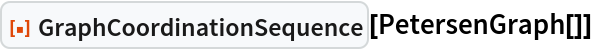Out=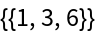Show the GraphDistanceMatrix in unsorted and sorted form:

 In:=Out=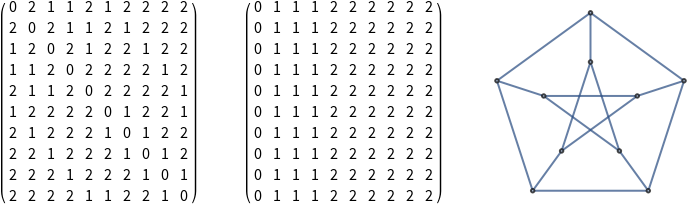Construct the order-7 middle levels graph (here, the middle levels are 3 and 4):

 In:=Out=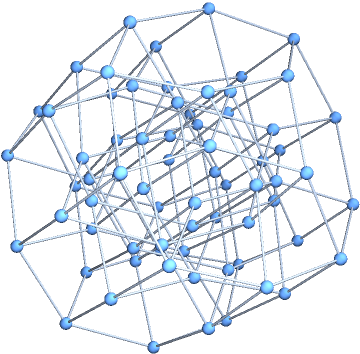Find the coordination sequence:

 In:=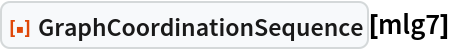Out=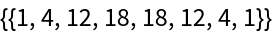The coordination sequence for any odd order middle levels graph can be found with a formula:

 In:=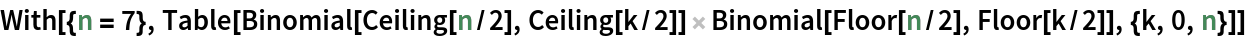Out=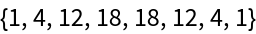Find the coordination sequence for a disconnected graph:

 In:=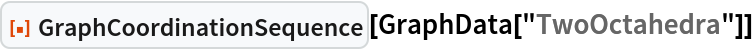Out=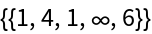### Scope (3)

Directed graphs may be used, such as seen in CayleyGraph.

The following graph is Fibonacci to ten steps in the coordination sequence:

 In:=Out=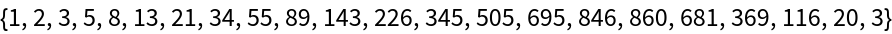The following graph is tribonacci to ten steps in the coordination sequence:

 In:=Out=The following graph is Padovan to ten steps in the coordination sequence:

 In:=Out=In:=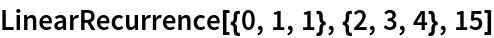Out=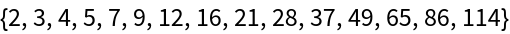### Properties and Relations (2)

The coordination sequences of hypercube graphs are binomial:

 In:=Out=The coordination sequences of the Bruhat graphs are the Mahonian numbers, A008302:

 In:=Out=### Possible Issues (4)

Multiple graphs can have the same coordination sequence:

 In:=Out=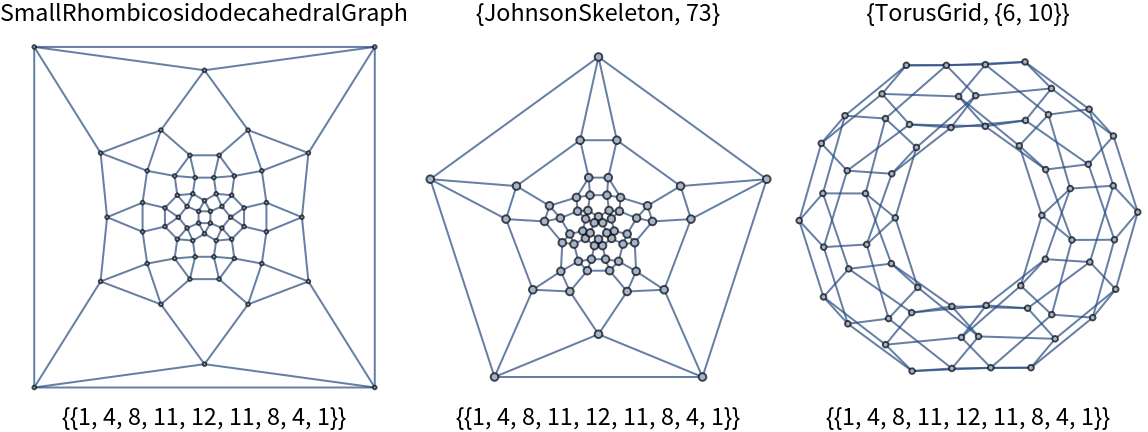Some graphs show different distant behaviors for every vertex:

 In:=Out=The coordination sequence for this graph has maximal dis-coordination:

 In:=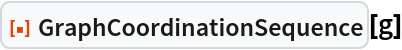Out=Up to 9 vertices, all regular connected graphs have the same coordination sequence for all vertices, with two exceptions:

 In:=Out=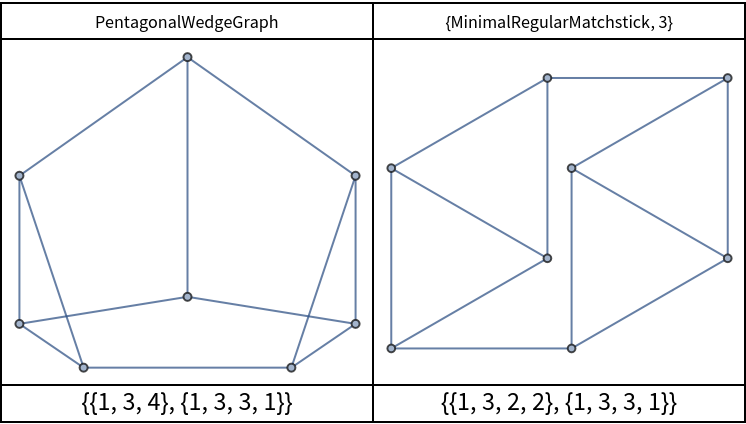Graphs with a specific coordination sequence are hard to find. Here are several of the form {1,3,6,x,x,4}:

 In:=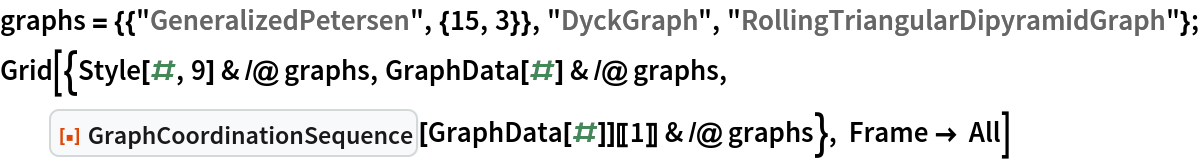Out=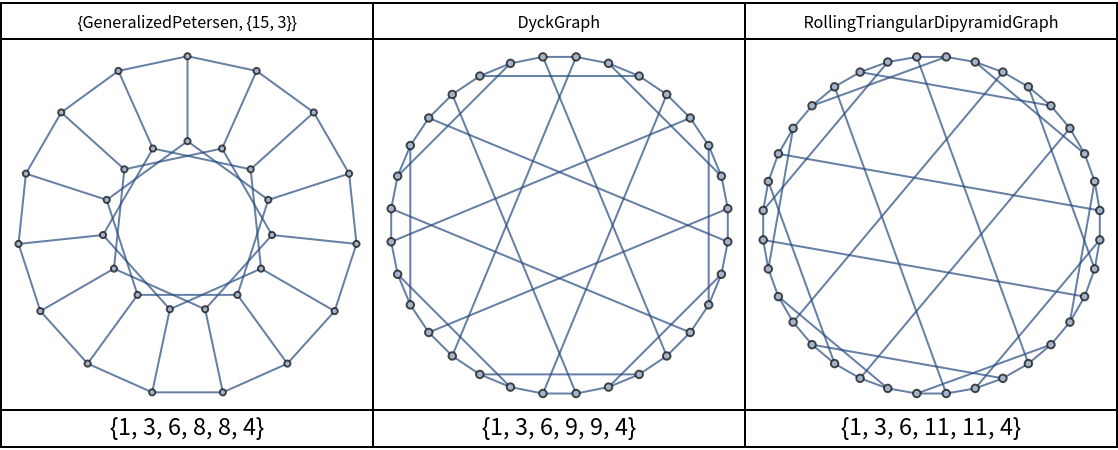Determining the existence of all {1,3,6,10,10,4} graphs currently requires a search of the 453090162062723 cubic graphs on 34 nodes.

### Neat Examples (2)

Here are 947 Cayley graphs with precomputed {order, coordination sequence, generator}:

 In:=Out=Coordination sequences have a rise followed (usually) by a fall:

 In:=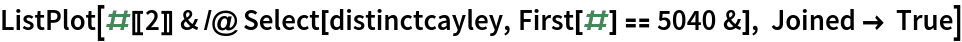Out=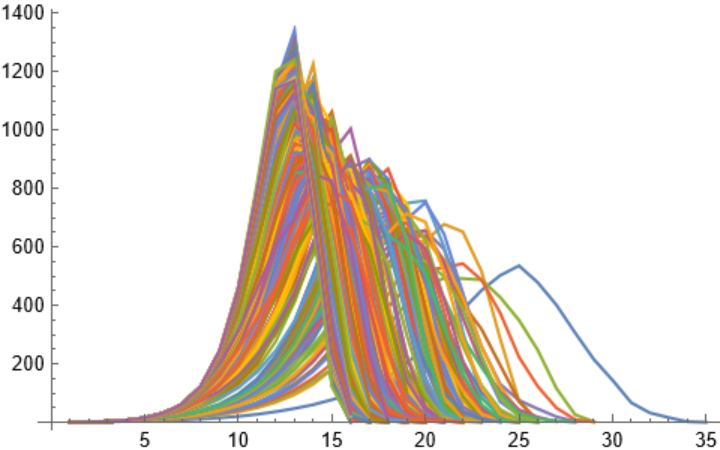## Version History

• 1.0.0 – 11 October 2022